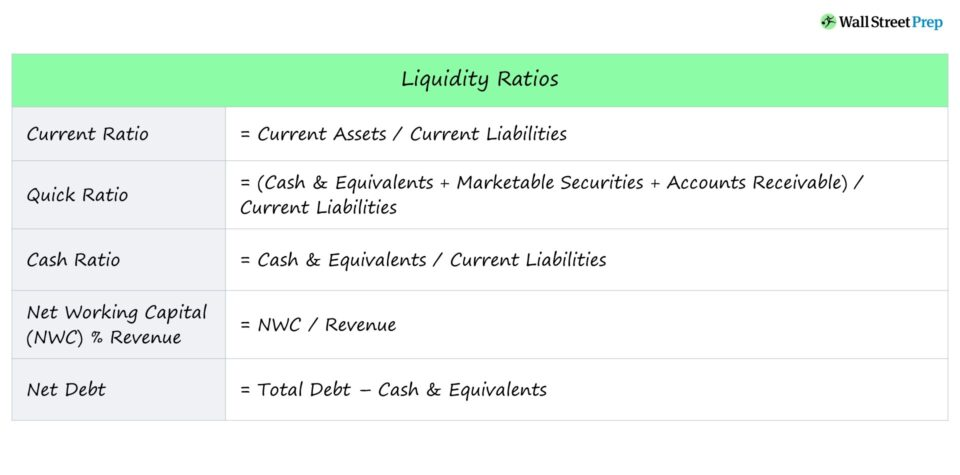# Liquidity Ratio

Guide to Understanding the Concept of Liquidity Ratios## How to Calculate Liquidity Ratios

Liquidity is defined as how quickly an asset can be converted into cash – so assets that can be sold and turned into cash in a short amount of time are considered to be highly liquid (and vice versa for assets with low liquidity).

Other than cash itself, assets with the highest liquidity include:

• Treasury Bills and Treasury Bonds
• Certificate of Deposits (CDs)
• Marketable Securities
• Short-Term Investments
• Money Market Funds

Other assets considered to be highly liquid are:

However, the actual liquidity of these assets tends to be dependent on the company.

Accounts receivable, the uncollected payments from customers that paid on credit, are not guaranteed to be received (i.e. “bad A/R”) and can be time-consuming to collect.

As for inventory, finding interested buyers can require steep discounts, so the sale price is often lower than the value as stated on the books (or could even remain unsold).

With that said, liquidity ratios can come in various forms, but the most common are as follows.

The pattern among each of these measures of liquidity is the short-term focus and the amount of value placed on current assets (rather than current liabilities).

## Liquidity Ratio #1 — Current Ratio Formula

The current ratio measures a company’s capacity to pay off all its short-term obligations.

The current ratio includes all current assets that can be converted into cash within one year and all current liabilities with maturities within one year.

Generally, a current ratio around 1.5x to 3.0x is considered “healthy,” with a current ratio of <1.0x being a sign of impending liquidity problems.

###### Current Ratio Formula
• Current Ratio = Current Assets / Current Liabilities

## Liquidity Ratio #2 — Quick Ratio Formula

The quick ratio is a more stringent variation of the current ratio, including only the most liquid assets – or more specifically, assets that can be converted into cash within 90 days with a high degree of certainty.

While dependent on the specific industry, the quick ratio should generally exceed >1.0x.

###### Quick Ratio Formula
• Quick Ratio = (Cash & Equivalents + Marketable Securities + Accounts Receivable) / Current Liabilities

## Liquidity Ratio #3 — Cash Ratio Formula

Of the ratios listed thus far, the cash ratio is the most conservative measure of liquidity.

The cash ratio measures a company’s ability to meet short-term obligations using only cash and cash equivalents (e.g. marketable securities).

If the cash ratio equals 1.0x, the company has exactly enough cash and cash equivalents to pay off short-term liabilities, so anything higher would be considered a positive sign (i.e. cash & equivalents are adequate).

###### Cash Ratio Formula
• Cash Ratio = Cash & Equivalents / Current Liabilities

## Liquidity Ratio #4 — Net Working Capital % Revenue Formula

Net working capital (NWC) is equivalent to current operating assets (i.e. excluding cash & equivalents) less current operating liabilities (i.e. excluding debt and debt-like instruments).

The NWC metric indicates whether a company has cash tied up within operations or sufficient cash to meet its near-term working capital needs.

• Positive NWC ➝ More Cash is Tied Up in Operations, Less Free Cash Flows
• Negative NWC ➝ Less Cash is Tied Up in Operations, More Free Cash Flows

With that said, from a liquidity standpoint, a negative NWC is preferred over a positive NWC.

There is no set percentage that all companies strive for, as the optimal level of NWC is dependent on the company’s specific industry and business model, but higher ratios are typically perceived negatively.

Higher NWC/Revenue ratios mean that more current assets are locked up in the business, which reduces liquidity – e.g. the company is struggling to collect cash payments from customers that paid on credit or facing difficulty in selling off inventory.

###### Net Working Capital % Revenue Formula
• NWC % Revenue = NWC / Revenue

## Liquidity Ratio #5 — Net Debt Formula

The net debt metric measures how much of a company’s short-term and long-term debt obligations could be paid off right now with the amount of cash available on its balance sheet.

Note that net debt is not a liquidity ratio (i.e. includes long-term debt) but is still a useful metric to evaluate a company’s liquidity.

If two identical companies have the same amount of debt in their capital structure – but one has a lower net debt balance – it can be implied that this company has comparatively more liquidity (and reduced risk).

###### Net Debt Formula
• Net Debt = Total Debt – Cash & EquivalentsStep-by-Step Online Course

#### Everything You Need To Master Financial Modeling

Enroll in The Premium Package: Learn Financial Statement Modeling, DCF, M&A, LBO and Comps. The same training program used at top investment banks.Inline FeedbacksLearn Financial Modeling Online

Everything you need to master financial and valuation modeling: 3-Statement Modeling, DCF, Comps, M&A and LBO.

X

The Wall Street Prep Quicklesson Series

7 Free Financial Modeling Lessons

Get instant access to video lessons taught by experienced investment bankers. Learn financial statement modeling, DCF, M&A, LBO, Comps and Excel shortcuts.